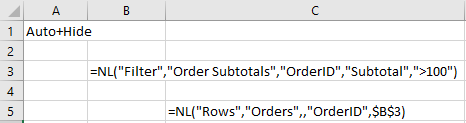Community
Training
Submit a Request
Become a Jet Insider
Give Feedback

# Using NL(Filter)

Beginner

Related Articles...

The NL(Filter) function returns a string value that can be used as a filter in another NL function.  Its intended use is for filtering the contents of one table based on the contents of another table.

## Example of the NL(Filter) function

#### The following formula will retrieve a list of records from the Orders table:

`=NL("Rows","Order")`

NL(Filter) can be used to filter on a field that is not on the Orders table (such as the "Subtotal" field on the “Order Subtotals” table):

`=NL("Rows","Orders",,"OrderID",NL("Filter","Order Subtotals","OrderID","Subtotal",">100"))`

The inner NL(Filter) function returns an array of the values of the OrderID field where the value of the Subtotal field is greater than 100.  The Jet Excel add-in can use this array as a filter for the Orders table.

The NL(Filter) function can also be placed in a separate cell and referenced by the NL(Rows) function: